#Function Repository Resource:

# ZeckendorfRepresentation

Give the 0–1 list that indicates the unique nonconsecutive Fibonacci numbers that sum to the non-negative integer input

Contributed by: Wolfram Staff
 ResourceFunction["ZeckendorfRepresentation"][n] gives the 0–1 list that indicates the unique nonconsecutive Fibonacci numbers that sum to the non-negative integer n.

## Details and Options

The Fibonacci numbers here are considered to be 1, 2, 3, 5, …, not 1, 1, 2, 3, 5, …; otherwise, the representation would not be unique.

## Examples

### Basic Examples

The first number whose representation takes three summands is 12:

 In:=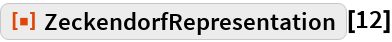Out=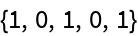This corresponds to 8 + 3 + 1:

 In:=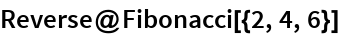Out=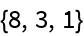The first number whose representation takes four summands is 33:

 In:=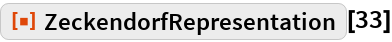Out=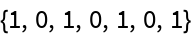In:=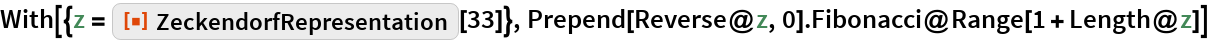Out=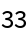### Neat Examples

 In:=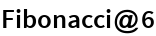Out=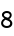There are Fk Zeckendorf representations of length k; for example, here are the 13 representations of length 7:

 In:=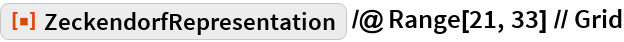Out=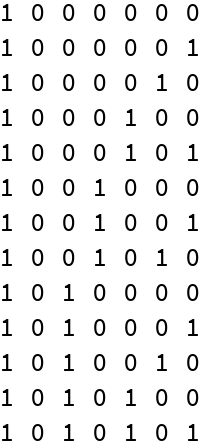This visualizes the same pattern:

 In:=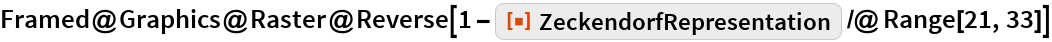Out=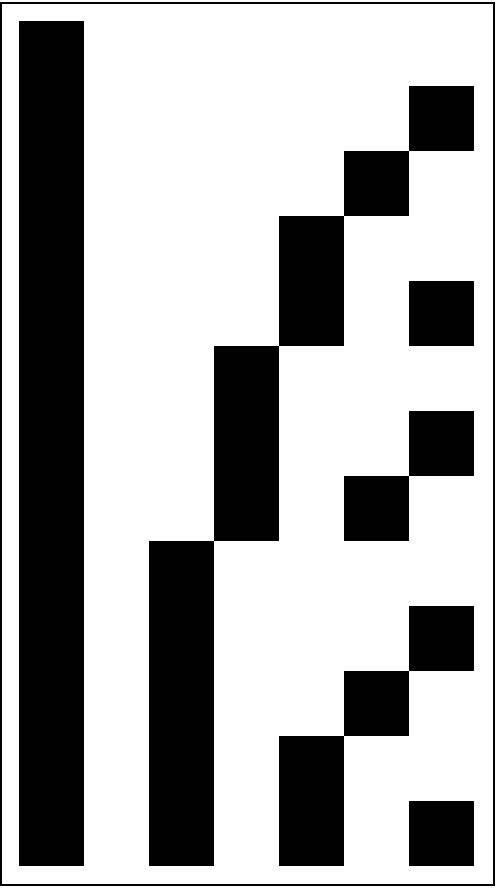## Requirements

Wolfram Language 11.3 (March 2018) or above